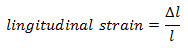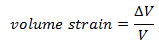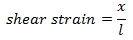# Strain and Hooke's Law<

### 3. Strain

• When a body is under a system of forces or couples in equilibrium then a change is produced in the dimensions of the body.
• This fractional change or deformation produced in the body is called strain.
• Strain is a dimensionless quantity.
• Strain is of three types
(a) Longitudinal strain:- It is defined as the ratio of the change in length to the original length. If l is the original length and Δl is the change in length then,(b) Volume strain:-It is defined as the ratio of change in volume to the original volume(c) Shearing strain:- If the deforming forces produce change in shape of the body then the strain is called shear strain. Considering Figure 2. it can also be defined as the ratio of displacement x of corner b to the transverse dimension l. Thusor,
Shear strain = tanθ
In practice since x is much smaller than l so, tanθ ≅ θ and the strain is simply the angle θ(measured in radians). Thus, shear strain is pure number without units as it is ratio of two lengths.
What is elastic limit> Elastic limit is the upper limit of deforming force up to which , if deforming force is removed, the body regains its original form completely beyond which if deforming force is increased, the body looses its property of elasticity and gets permanently deformed.

### 4. Hook's Law

• Hook's law is the fundamental law of elasticity and is stated as " for small deformations stress is proportional to strain".
Thus,
stress ∝ strain
or,
$\frac{stress}{strain}=constant$
This constant is known as modulus of elasticity of a given material, which depends upon the nature of the material of the body and the manner in which body is deformed.
• Hook's law is not valid for plastic materials.
• Units and dimension of the modulus of elasticity are same as those of stress.

This mobile-friendly simulation allows students to stretch and compress springs to explore relationships among force, spring constant, displacement, and potential energy in a spring. You can use it to promote understanding of the predictable mathematical relationships that underlie Hooke's Law. Playing around with this simulation you can get an understanding of restoring forces.